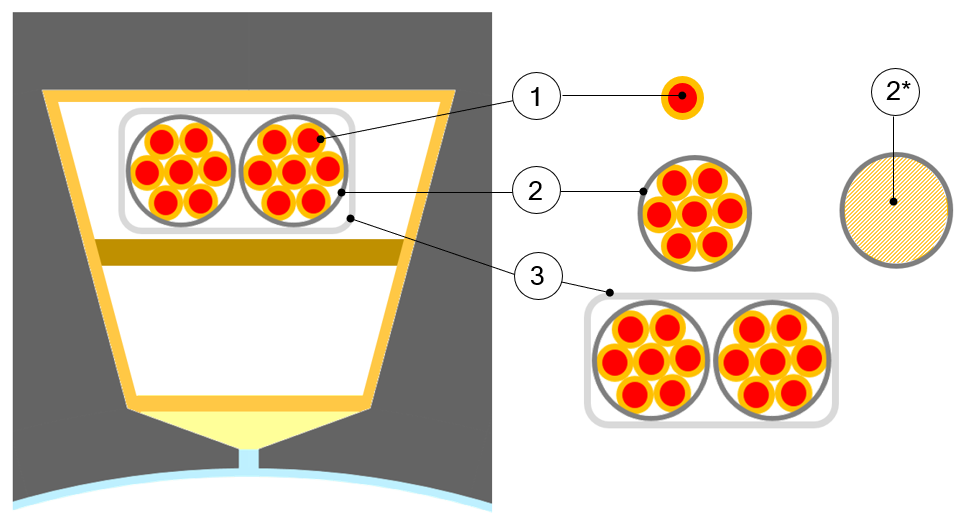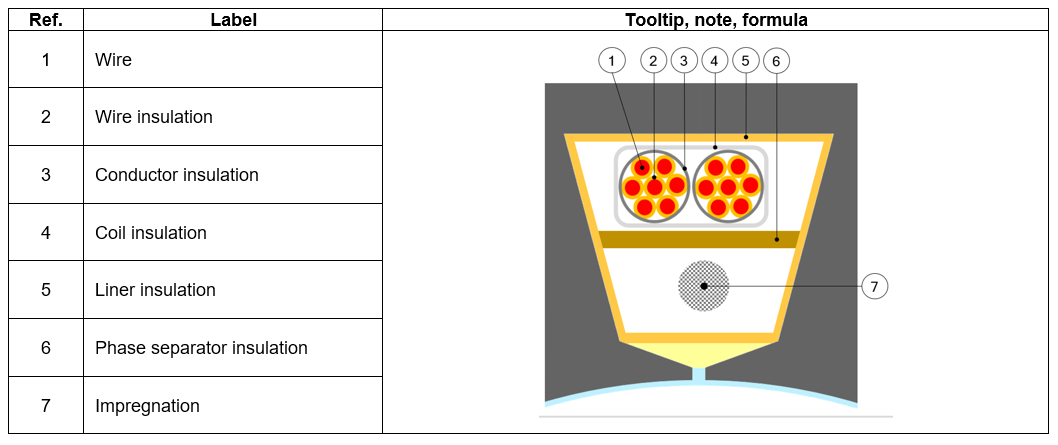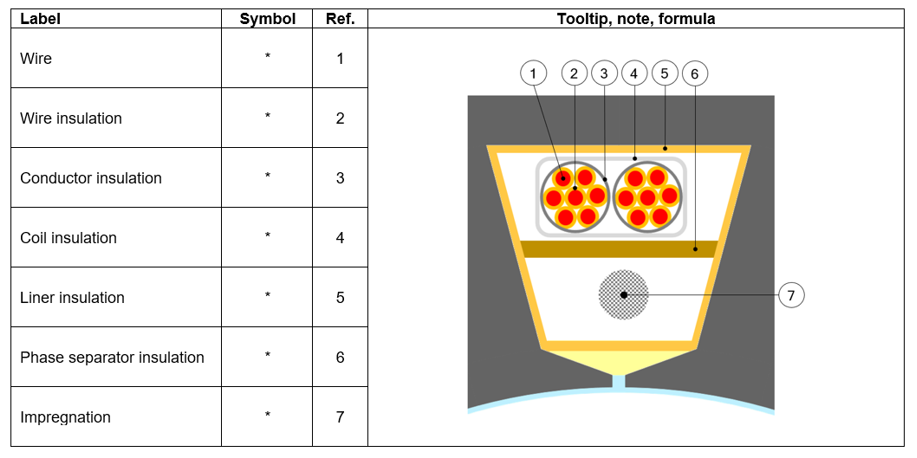# Winding outputs

## 2. Definition – illustrationSlot composition 1 Wire also called strand. 2 Conductor. That also corresponds to a turn section (one conductor = one turn).A conductor is composed with one or several wires in parallel. 2* The hatched area corresponds to the conductor’s useful area. Area which includes: the wires + insulation + free space. This is not the conductive area. 3 Coil which is an assembly of several conductors (i.e. several turns per coil).

## 3. Inputs

 Label Symbol Tooltip, note, formula Winding connection Connect Winding connection (Y – Wye or Δ - Delta) Definition mode * Winding definition mode: Automatic, Easy, Advanced or Expert. No. parallel paths P paths Number of parallel paths (all modes). Phase sequence * Phase sequence (all modes).

## 4. Settings – Coil – Conductor

 Label Symbol Tooltip, note, formula No. turns per coil Turns Number of turns per coil. No. wires in hand No.Wires Number of wires in parallel in a conductor (per turn) i.e. number of wires in parallel in each conductor. Wire topology * Wire topology – Circular or Rectangular. Wire diameter ∅ wire Wire diameter (without insulation). Wire width * For rectangular shape type wire. Wire height * For rectangular shape type wire. Conductor topology * Cable topology – Circular or RectangularCoil conductors are grouped inside a circular shape or a rectangular shape. It is linked to the wire topology.Rectangular wires impose a rectangular cable. Circular wires impose a circular cable. Conductor diameter * Cable diameter (without cable insulation) i.e. Diameter of the circle which are grouped inside all the coil conductors. Conductor width * Cable width (without conductor insulation) i.e. Width of the rectangle which are grouped inside all the coil conductors - Case of rectangular shape type wire. Conductor height * Cable height (without conductor insulation) i.e. Height of the rectangle which are grouped inside all the coil conductors - Case of rectangular shape type wire.

## 5. Setting – Coil Insulation thickness

For more details see section “Choice of winding MATERIALS”. All the thicknesses are defined as user input parameters in winding – Settings – Coil tab.

 Label Symbol Tooltip, note, formula Wire * Insulation thickness of the wire Conductor * Insulation thickness of the conductor Coil * Insulation thickness of the coil Liner * Insulation thickness of the liner Phase separator * Insulation thickness of the phase separator Impregnation * Insulation spread inside the slot Impregnation goodness * Quality of impregnation (percentage of winding impregnation)## 6. Settings – Coil – End winding

This part characterizes the end-winding and the resulting conductor dimensions.

For additional information refer to the chapter dedicated to the coil and conductor settings - End-winding topology and dimensions.

 Label Symbol Tooltip, note, formula End-winding topology * End-winding topology: U-shape, C-shape or Y-shape. C.S. total extension * Connection side total extension. C.S. straight extension * Connection side straight extension Axial overall length * Axial overall length. Length between the two extremities of the winding i.e. between connection side and opposite connection side. O.C.S. total extension * Opposite connection side total extension. O.C.S. straight extension * Opposite connection side straight extension. Total conductor length * Total conductor length. Mean turn length * Mean turn length. Coil connection length * Additional length corresponding to the connections between coils.

## 7. Settings – Calibration factors

 Label Symbol Tooltip, note, formula Resistance factor * Setting of the “Resistance factor”. It allows adjusting computation result of resistance with resistance measurement. Thus, the resulting phase resistance value is considered wherever it is. Inductance factor * Setting of the “Inductance factor”. It allows modifying the computation result of end-winding inductance. Thus, the resulting end-winding inductance value is considered wherever it is. Ref. temperature * The reference temperature.First, resistance values are always computed by considering a temperature equal to 20°C. However the user can also define his own reference temperature to compute the corresponding phaseresistance and Line-Line resistance values.Caution : This reference temperature is used only in the winding design environment. The test temperatures are defined in the test settings (refer to TEST chapter).

## 8. Materials

This part characterizes the end-winding and the resulting conductor dimensions.

For additional information refer to the chapter dedicated to the coil and conductor settings - End-winding topology and dimensions.